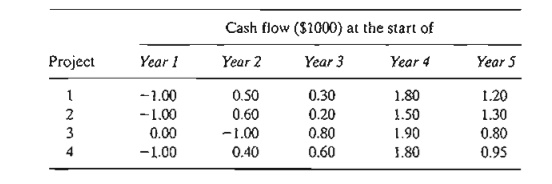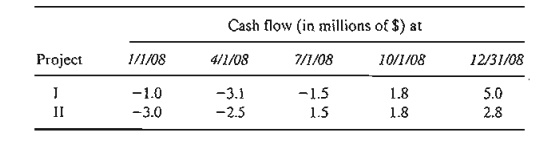Home | | Resource Management Techniques | Selected LP Applications: Investment

# Selected LP Applications: Investment

Today's investors are presented with multitudes of investment opportunities. Examples of investment problems are capital budgeting for projects, bond investment strate-gy, stock portfolio selection, and establishment of bank loan policy.

SELECTED LP APPLICATIONS

This section presents realistic LP models in which the definition of the variables and the construction of the objective function and constraints are not as straight-forward as in the case of the two-variable model. The areas covered by these appli-cations include the following:

1. Urban planning.

2. Currency arbitrage.

3. Investment.

4. Production planning and inventory control.

5. Blending and oil refining.

6. Manpower planning.

Each model is fully developed and its optimum solution is analyzed and interpreted.

Investment

Today's investors are presented with multitudes of investment opportunities. Examples of investment problems are capital budgeting for projects, bond investment strate-gy, stock portfolio selection, and establishment of bank loan policy. In many of these situations, linear programming can be used to select the optimal mix of opportunities that will maximize return while meeting the investment conditions set by the investor.

Example 2.3-3   (Loan Policy Model)

TIlfiftem Bank is in the process of devising a loan policy that involves a maximum of \$12 million. The following table provides the pertinent data about available types of loans.Bad debts are unrecoverable and produce no interest revenue.

Competition with other financial institutions requires that the bank allocate at least 40% of the funds to farm and commercial loans. To assist the housing industry in the region, home loans must equal at least 50% of the personal, car, and home loans. TIle bank also has a stated policy of not allowing the overall ratio of bad debts on all loans to exceed 4%.

Mathematical Model: The situation seeks to determine the amount of loan in each category, thus leading to the following definitions of the variables:

xi = personal loans (in millions of dollars)

x2 =  car loans

x3 = home loans

x4 = farm loans

x5commercial loans

The objective of the Thriftem Bank is to maximize its net return, the difference between interest revenue and lost bad debts. The interest revenue is accrued only on loans in good standing. Thus, because 10% of personal loans are lost to bad debt, the bank will receive interest on only 90% of the loan-that is, it will receive 14% interest on .9x( of the original loan XI' The same reasoning applies to the remaining four types of loans. Thus,The problem has five constraints:

1. Total funds should not exceed \$12 (million):2. Farm and commercial loans equal at least 40% ofall loans:3. Home loans should equal at least 50% of personal, car, and home loans:4. Bad debts should not exceed 4% ofall loans:5.    Nonnegalivily:A subtle assumption in the preceding formulation is that all loans are issued at approxi-mately the same time. 'This assumption allows us 10 ignore differences in the time value of the funds allocated to the different loans.

Solution:

The optimal solution is

z = .99648, xl  = 0, x2  = 0, x3  = 7.2, x4  = 0, x5  =  4.8

Remarks.

1. You may be wondering why we did not define the right-hand side of the second constraint as.4 x 12 instead of .4(x1 + x2 + x3 + x4 + x5). After all, it seems logical that the bank would want to loan out all \$12 (million). The answer is that the second usage does not "rob" the model of this possibility. If the optimum solution needs all \$12 (million), the given con-straint will allow it. But there are two important reasons why you should not use .4 X 12: (1) If other constraints in the model are such that all \$12 (million) cannot be used (for example, the bank may set caps on the different loans), then the choice .4 X 12 could lead to an infeasible or incorrect solution. (2) If you want to experiment with the effect of changing available funds (say from \$12 to \$13 million) on the optimum solution, there is a real chance that you may forget to change.4 x 12 to.4 x 13, in which case the solution you get will not be correct. A similar reasoning applies to the left-hand side of the fourth constraint.

2. The optimal solution calls for allocating all \$12 million: \$7.2 million to home loans and \$4.8 million to commercial loans. The remaining categories receive none. The return on the investment is computed asThis shows that the combined annual rate of return is 8.034 %, which is less than the best net interest rate (= .0864 for home loans), and one wonders why the optimum does not . take advantage of this opportunity. The answer is that the restriction stipulating that farm and commercial loans account for at least 40% of all loans (constraint 2) forces the solution to allocate \$4.8 million to commercial loans at the lower /let rate of .078, hence lowering the overall interest rate to [.0864 x 7.2 + .078 x 4.8 ]/ 12= .08034. In fact, if we remove constraint 2, the optimum will allocate all the funds to home loans at the higher 8.64% rate.

PROBLEM SET 2.3C

1. Fox Enterprises is considering six projects for possible construction over the next four years. The expected (present value) returns and cash outlays for the projects are given below. Fox can undertake any of the projects partially or completely. A partial undertak-ing of a project will prorate both the return and cash outlays proportionately.a.Formulate the problem as a linear program, and determine the optimal project mix that maximizes the total return. Ignore the time value of money.

b.Suppose that if a portion of project 2 is undertaken then at least an equal portion of project 6 must undertaken. Modify the formulation of the model and find the new optimal solution.

c.In the original model, suppose that any funds left at the end of a year are used in the next year. Find the new optimal solution, and determine how much each year "bor-rows" from the preceding year. For simplicity, ignore the time value of money.

d.Suppose in the original model that the yearly funds available for any year can be ex-ceeded, if necessary, by borrowing from other financial activities within the company. Ignoring the time value of money, reformulate the LP model, and find the optimum solution. Would the new solution require borrowing in any year? If so, what is the rate of return on borrowed money?

2. Investor Doe has \$10,000 to invest in four projects. The following table gives the cash flow for the four investments.The information in the table can be interpreted as follows: For project 1, \$1.00 invested at the start of year 1 will yield \$.50 at the start of year 2, \$.30 at the start of year 3, \$1.80 at the start of year 4, and \$1.20 at the start of year 5. The remaining entries can be interpreted similarly. The entry 0.00 indicates that no transaction is taking place. Doe has the additional option of investing in a bank account that earns 6.5% annually. All funds ac-cumulated at the end of one year can be reinvested in the following year. Formulate the problem as a linear program to determine the optimal allocation of funds to investment opportunities.

3. Hi Rise Construction can bid on two I-year projects. The following table provides the quarterly cash flow (in milIions of dollars) for the two projects.HiRise has cash funds of \$1 million at the beginning of each quarter and may borrow at most \$1 million at a 10% nominal annual interest rate. Any borrowed money must be re-turned at the end of the quarter. Surplus cash can earn quarterly interest at an 8% nomi-nal annual rate. Net accumulation at the end of one quarter is invested in the next quarter.

a. Assume that HiRise is allowed partial or full participation in the two projects. De-termine the level of participation that will maximize the net cash accumulated on 12/31/2008.

b. Is it possible in any quarter to borrow money and simultaneously end up with sur-plus funds? Explain.

4. In anticipation of the immense college expenses, a couple have started an annual investment program on their child's eighth birthday that will last until the eighteenth birthday. The couple estimate that they will be able to invest the following amounts at the beginning of each year:To avoid unpleasant surprises, they want to invest the money safely in the following options: Insured savings with 7.5% annual yield, six-year government bonds that yield 7.9% and have a current market price equal to 98% of face value, and nine-year municipal bonds yielding 8.5% and having a current market price of 1.02 of face value. How should the couple invest the money?

5. A business executive has the option to invest money in two plans: Plan A guarantees that each dollar invested will earn \$.70 a year later, and plan B guarantees that each dollar invested will earn \$2 after 2 years. In plan A, investments can be made annually, and in plan B, investments are allowed for periods that are multiples of two years only. How should the executive invest \$100,000 to maximize the earnings at the end of 3 years?

6. A gambler plays a game that requires dividing bet money among four choices. The game has three outcomes. The following table gives the corresponding gain or loss per dollar for the different options of the game.The gambler has a total of \$500, which may be played only once. The exact outcome of the game is not known a priori. Because of this uncertainty, the gambler's strategy is to maximize the minimum return produced by the three outcomes. How should the gambler allocate the \$500 among the four choices? (Hint:The gambler's net return may be positive, zero, or negative.)

7. (Lewis, 1996) Monthly bills in a household are received monthly (e.g., utilities and home mortgage), quarterly (e.g., estimated tax payment), semiannually (e.g., insurance) ,or annually (e.g., subscription renewals and dues). The following table provides the monthly bills for next year.To account for these expenses, the family sets aside \$1000 per month, which is the average of the total divided by 12 months. If the money is deposited in a regular savings account, it can earn 4% annual interest, provided it stays in the account at least one month. The bank also offers 3-month and 6-month certificates of deposit that can earn 5.5% and 7% annual interest, respectively. Develop a 12-month investment schedule that will maximize the family's total return for the year. State any assumptions or requirements needed to reach a feasible solution.

Study Material, Lecturing Notes, Assignment, Reference, Wiki description explanation, brief detail
Operations Research: An Introduction : Modeling with Linear Programming : Selected LP Applications: Investment |